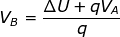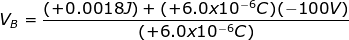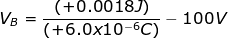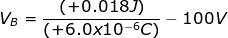0
733

# Potential Energy: Electric Potential Formula

#### ∆U = UB – UA#### The charge is given in terms of micro-Coulombs (μC): 1.0 μC = 1.0 x 10-6C. The charge needs to be converted to the correct units before solving the equation:#### The electric potential at position B is +200 V.

READ HERE  Electrostatic Potential - Definition, SI Unit and Formula